Maths Puzzles

These tricky maths puzzles are a fun way for 3rd and 4th graders to practise arithmetic and algebraic thinking.

Click on a worksheet in the set below to see more info or download the PDF.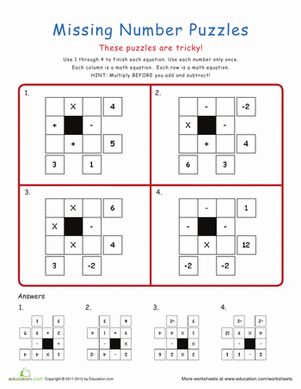Maths Puzzles

Calling all Sudoku and Ken Ken buffs! These maths puzzles are tricky, but are a fun and simpler spin-off of the popular Ken Ken puzzle.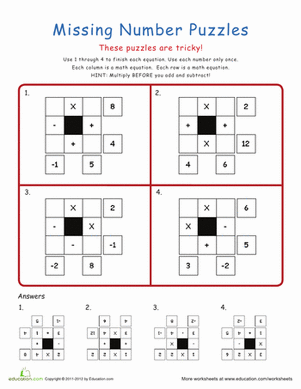Missing Number maths Puzzles

Calling all Sudoku and Ken Ken buffs! Give your student a fun way to practise maths and problem solving with these fun puzzles.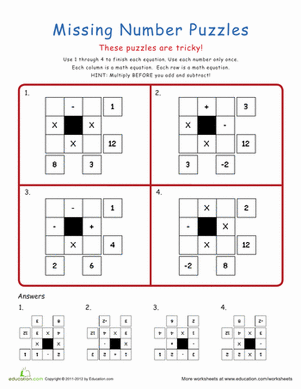Maths Puzzlers

Hey maths buffs, do you need a challenge? These maths puzzles are tricky, but are a fun and simpler spin-off of the Ken Ken puzzle.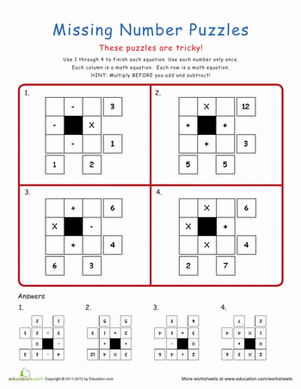Tricky maths Puzzles

Is your child a maths whiz who needs a challenge? These maths puzzles are tricky, but are a fun and simpler spin-off of the Ken Ken puzzle.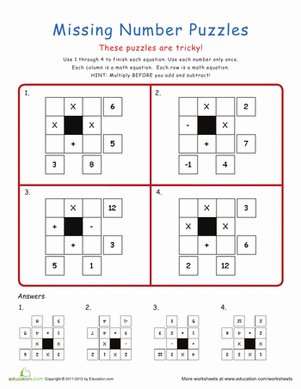Fun Fun maths Puzzles

Fun Fun puzzles are almost like Ken Ken! These ones are tricky, but very fun to solve. Give your student a fun way to practise simple arithmetic.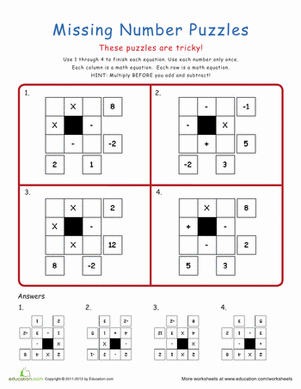Maths Grid Puzzles

Calling all Sudoku and Ken Ken buffs! These maths puzzles are tricky, but are a fun and simpler spin-off of the Ken Ken puzzle.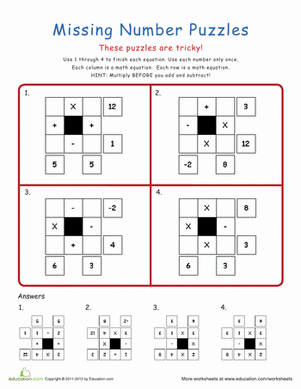Four maths Puzzles

This is for all you maths whizzes who need a challenge! These maths puzzles are tricky, but are a fun and simpler spin-off of the Ken Ken puzzle.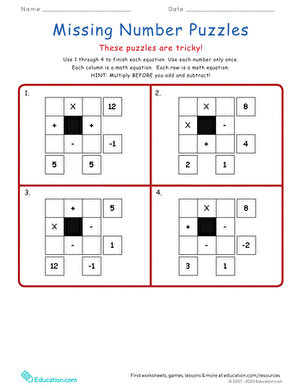Maths Puzzle Mania!

Maths maniacs, these challenging puzzles are for you! These four puzzles are tricky, but are a fun and simpler spin-off of the Ken Ken puzzle.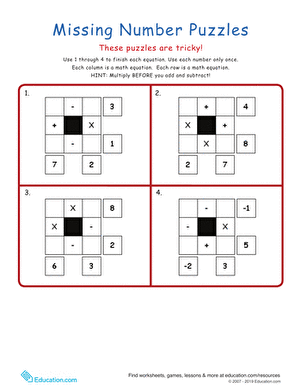If your child is mad for maths, give him a challenge! These maths puzzles are tricky, but are a fun and simpler spin-off of the Ken Ken puzzle.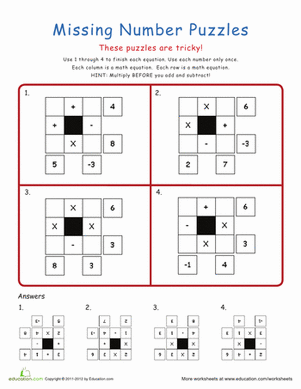Amazing maths Puzzles

This missing numbers maths puzzle will get your kid thinking creatively. Kids who enjoy Ken Ken and Sudoku puzzles will like this amazing maths puzzle.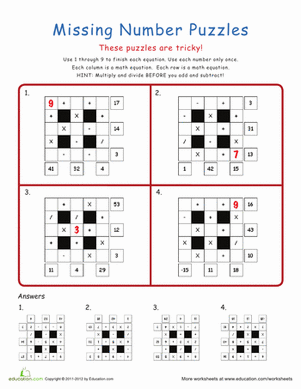Maths Puzzles: Intermediate

If your child has a knack for number games, he'll love this mysterious maths puzzle. Kids will use addition, subtraction, multiplication, and division skills.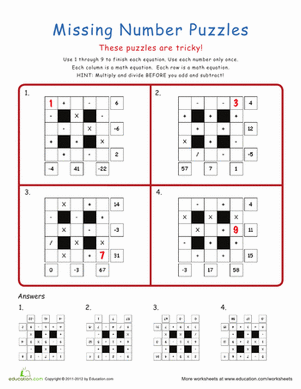Maths Mania Puzzles

Looking for a way to get your child excited about maths? Try these maths puzzles, a tricky but fun spin-off of the popular Ken Ken puzzle!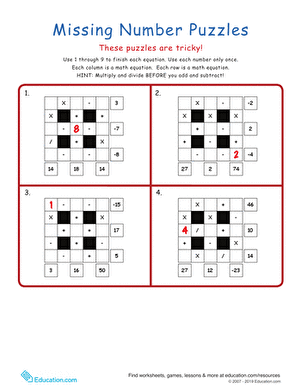Crazy maths Puzzles

Hey maths whizzes, here is a challenge for you! These maths puzzles are tricky, but are a fun spin-off of the popular Ken Ken puzzle.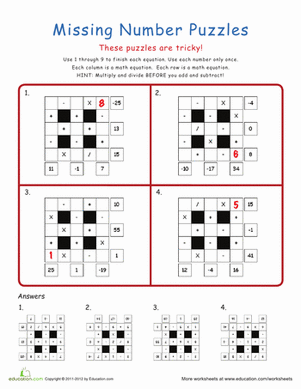Great Grid maths Puzzles

Does your child need a little challenge in maths and problem solving? These maths puzzles are tricky, but are a fun spin-off of the popular Ken Ken puzzle.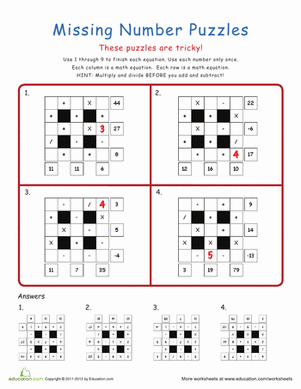Four Fantastic maths Puzzles

Calling all Sudoku and Ken Ken buffs, here's a little something new! These maths puzzles are tricky, but are a fun spin-off of the popular Ken Ken puzzle.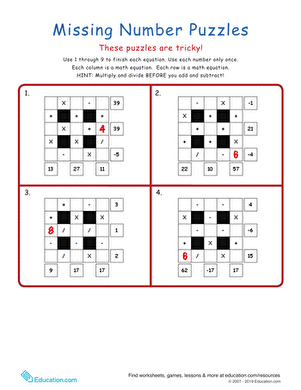Maths Grid Puzzlers

If your student needs a challenge in maths, try these maths puzzles! These maths grids are tricky, but are a fun spin-off of the popular Ken Ken puzzle.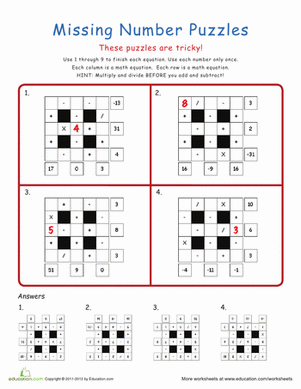More maths Puzzles

Looking for a way to get your child excited about maths? These maths puzzles are tricky, but are a fun spin-off of the popular Ken Ken puzzle.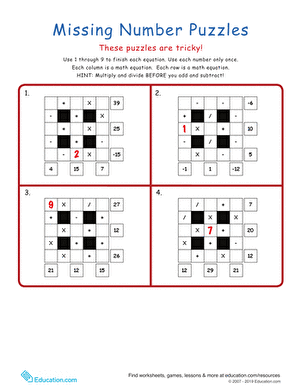Missing Number Puzzles

Calling all Sudoku and Ken Ken buffs, we have a new addicting puzzle for you! These are tricky, but a fun spin-off of the popular Ken Ken puzzle.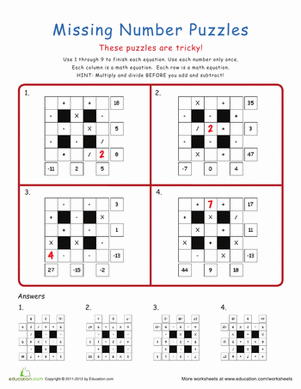Amazing maths Puzzlers

Keep your amazing maths student on his toes with some challenging puzzles! These maths grids are tricky, but are a fun spin-off of the popular Ken Ken puzzle.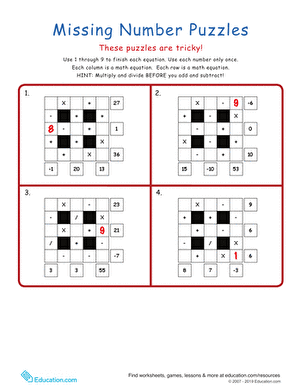Sudoku and Ken Ken enthusiasts will enjoy this mad maths puzzle, full of addition, subtraction, multiplication, and division challenges.

Want to download this whole set as a single PDF?

Create new collection

0

New Collection>

0Items

What could we do to improve Education.com?

Please note: Use the Contact Us link at the bottom of our website for account-specific questions or issues.

What would make you love Education.com?